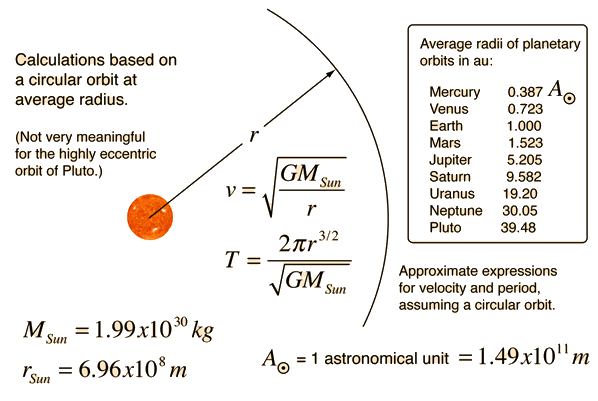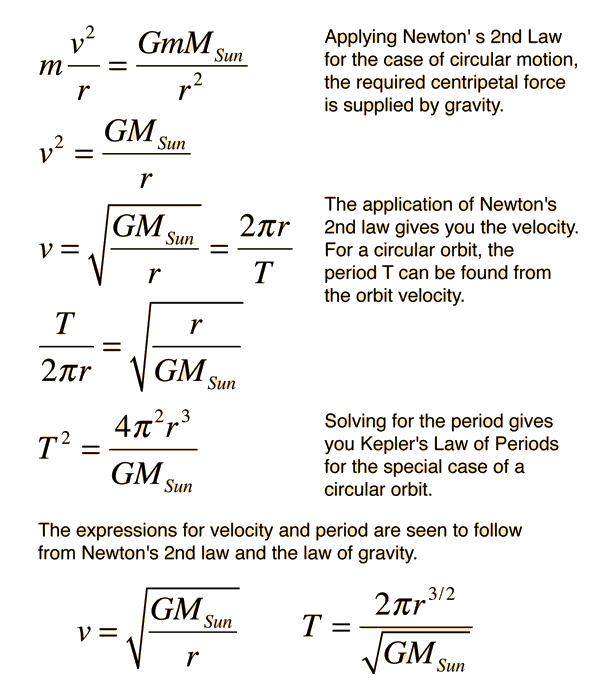# Solar OrbitPlanetary data

Note: The orbit radii in this table are from the NASA Solar System Data Table.

Accurate values for the periods of the planets can be calculated from Kepler's Law of Periods and the semimajor axes of the elliptical orbits. But it may be instructive to calculate approximate values for the periods and orbital velocities assuming a circular orbit at the average orbital radius.

 If the average orbital radius r = rEarth

 then the approximate period T = Earth days
T = Earth years.
 and the approximate orbital velocity is vorbit = km/s

vorbit =km/hr
vorbit =mi/hr

Note that the accurate length of the Earth year is 365.256 days, so approximating the Earth's orbit with a circle gives an error of about 2 days or about 0.6%, so the Earth's orbit is very close to being a circle. The departure from circular orbit for the planets can be expressed in terms of the eccentricity of the orbits.

These approximations make use of Newton's second law, the law of universal gravitation and the concept of centripetal force. A perfectly circular orbit can be precisely described as follows:Index

Gravity concepts

Orbit concepts

 HyperPhysics***** Mechanics ***** Rotation R Nave
Go Back